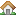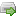•Home
•Demo
•Downloads
•Manual
•Support Forum
You are here:  » Record Pagination

# Record Pagination

Submitted by jayt43 on Sat, 2008-08-09 18:19 in

Hi David,

Having bought Magic Parser yesterday, I'm currently trying to get the same functionality from a feed as in your demo i.e. one record is shown per page, with a next/previous link to navigate between records.

Having checked out the examples on this website, I came across: http://www.magicparser.com/node/414 which is one of several that include such functionality. However, having topped and tailed the code for my feed, all the records from it are listed on just the one page. I can navigate forwards/backwards, but all of the same records are shown again.

Here's the feed in question:

http://www.affiliate.viator.com/xml/affiliateXMLData.jsp?IATA=BUD

So basically, I'm trying to put each ProductItem on a different page.

Here's my code:

``` <?php//Get the file MagicParser.phprequire("MagicParser.php");if(!\$_GET['PAGE'])\$page = 1;// If there is a current page number, use it.else \$page = \$_GET['page'];//If there is no page number, set one!\$total_number_of_items = 0;//Count the total number of items in the xml feed\$items_per_page = 10;//Set the number of items displayed per page\$counter = 0;function myRecordCounter(\$record){global \$total_number_of_items;\$total_number_of_items++;// increment for each record in the feed}function myRecordHandler(\$item){global \$page;global \$items_per_page;global \$counter;\$counter++;// return false whilst parsing items on previous pagesif (\$counter <= ((\$page-1)*\$items_per_page)) return false;//Display the xml items that are to be displayed here as normalecho "<tr>    <td align=\"center\" width=\"200\" height=\"150\">        <a href='".\$item["EXAMPLE-ELEMENT"]."'><img src='".\$item["EXAMPLE-ELEMENT"]."' height='110' width='150' style='border-style: none' alt='hotel image' /></a>    </td>    <td width=\"410\" height=\"150\">        <p>        <strong>Example Text:</strong> <a href='".\$item["EXAMPLE-ELEMENT"]."'><strong>".\$item["EXAMPLE-ELEMENT"]."</strong></a>        </p>        <br />        <p>        <a href='".\$item["EXAMPLEITEM"]."'><strong>".\$item["EXAMPLE-ELEMENT"]."".\$item["EXAMPLE-ELEMENT"]."</strong> <em>example text</em></a>        <br />        </p>        <br />        <p>        ".\$item["EXAMPLE-ELEMENT"]."        </p>    </td></tr><tr>    <td align=\"center\" colspan=\"2\">    <span class=\"content-text-bold\">- - - - - - - - - - - - - - - - - - - - - - - - - - - - - - - - - - - - - - - - -</span>    </td></tr>";// return true if counter reaches current page * items on each pagereturn (\$counter == (\$page 1 \$items_per_page));}//Print the table head tagecho "<table class=\"content-text\" width=\"500\" border=\"0\" align=\"center\" cellpadding=\"0\" cellspacing=\"0\">";//Parse the xml feedsMagicParser_parse("http://www.affiliate.viator.com/xml/affiliateXMLData.jsp?IATA=BUD","myRecordCounter","xml|VIATORAFFILIATEXMLDATA/IATA/PRODUCTITEM/");MagicParser_parse("http://www.affiliate.viator.com/xml/affiliateXMLData.jsp?IATA=BUD","myRecordHandler","xml|VIATORAFFILIATEXMLDATA/IATA/PRODUCTITEM/");//Print the table foot tagecho "</table>";\$total_pages_count = (\$total_number_of_items/\$items_per_page);//Calculate the number of pages. Divide the total number of items by the number of items per page.\$total_number_of_pages = number_format(\$total_pages_count, 0);//Format the total number of pages so that decimals are not displayed//Display the current page number and the total number of pagesecho"<h1 align=\"center\">PAGE \$page of \$total_number_of_pages</h1>";    if (\$page==1){    //If the page number is 1 only display the Page 2 link    echo "<h1 align=\"center\"><a href='?page=".(\$page+1)."'>PAGE ".(\$page+1)." &gt;&gt;</a></h1>";    }    else{    //If the page number is 2 or higher, display the previous page number and the next page number links    echo"<h1 align=\"center\"><a href='?page=".(\$page-1)."'>&lt;&lt; PAGE ".(\$page-1)."</a> | <a href='?page=".(\$page+1)."'>PAGE ".(\$page+1)." &gt;&gt;</a></h1>";    }?> ```

So apologies if I'm doing something really wrong here as PHP is not my forte!

Many thanks Julian

Hello Julian,

I think it was just a typo at the top, where you have PAGE in capitals rather than lower case.

PHP's \$_GET variables are case sensitive.

I've fixed this in the following code, and also tidied up the Next / Prev so that they only
appear if there are are next and previous pages...

``` <?php//Get the file MagicParser.phprequire("MagicParser.php");if(!\$_GET['page'])\$page = 1;// If there is a current page number, use it.else \$page = \$_GET['page'];//If there is no page number, set one!\$total_number_of_items = 0;//Count the total number of items in the xml feed\$items_per_page = 10;//Set the number of items displayed per page\$counter = 0;function myRecordCounter(\$record){global \$total_number_of_items;\$total_number_of_items++;// increment for each record in the feed}function myRecordHandler(\$item){global \$page;global \$items_per_page;global \$counter;\$counter++;// return false whilst parsing items on previous pagesif (\$counter <= ((\$page-1)*\$items_per_page)) return false;//Display the xml items that are to be displayed here as normalecho "<tr>    <td align=\"center\" width=\"200\" height=\"150\">        <a href='".\$item["EXAMPLE-ELEMENT"]."'><img src='".\$item["EXAMPLE-ELEMENT"]."' height='110' width='150' style='border-style: none' alt='hotel image' /></a>    </td>    <td width=\"410\" height=\"150\">        <p>        <strong>Example Text:</strong> <a href='".\$item["EXAMPLE-ELEMENT"]."'><strong>".\$item["EXAMPLE-ELEMENT"]."</strong></a>        </p>        <br />        <p>        <a href='".\$item["EXAMPLEITEM"]."'><strong>".\$item["EXAMPLE-ELEMENT"]."".\$item["EXAMPLE-ELEMENT"]."</strong> <em>example text</em></a>        <br />        </p>        <br />        <p>        ".\$item["EXAMPLE-ELEMENT"]."        </p>    </td></tr><tr>    <td align=\"center\" colspan=\"2\">    <span class=\"content-text-bold\">- - - - - - - - - - - - - - - - - - - - - - - - - - - - - - - - - - - - - - - - -</span>    </td></tr>";// return true if counter reaches current page * items on each pagereturn (\$counter == (\$page * \$items_per_page));}//Print the table head tagecho "<table class=\"content-text\" width=\"500\" border=\"0\" align=\"center\" cellpadding=\"0\" cellspacing=\"0\">";//Parse the xml feedsMagicParser_parse("http://www.affiliate.viator.com/xml/affiliateXMLData.jsp?IATA=BUD","myRecordCounter","xml|VIATORAFFILIATEXMLDATA/IATA/PRODUCTITEM/");MagicParser_parse("http://www.affiliate.viator.com/xml/affiliateXMLData.jsp?IATA=BUD","myRecordHandler","xml|VIATORAFFILIATEXMLDATA/IATA/PRODUCTITEM/");//Print the table foot tagecho "</table>";\$total_pages_count = ceil(\$total_number_of_items/\$items_per_page);//Calculate the number of pages. Divide the total number of items by the number of items per page.\$total_number_of_pages = number_format(\$total_pages_count, 0);//Format the total number of pages so that decimals are not displayed//Display the current page number and the total number of pagesecho"<h1 align=\"center\">PAGE \$page of \$total_number_of_pages</h1>";    if ((\$page==1) && (\$total_number_of_pages > 1))    {    //If the page number is 1 only display the Page 2 link    echo "<h1 align=\"center\"><a href='?page=".(\$page+1)."'>PAGE ".(\$page+1)." &gt;&gt;</a></h1>";    }    elseif(\$page < \$total_number_of_pages)    {    //If the page number is 2 or higher and less than total number of pages    echo"<h1 align=\"center\"><a href='?page=".(\$page-1)."'>&lt;&lt; PAGE ".(\$page-1)."</a> | <a href='?page=".(\$page+1)."'>PAGE ".(\$page+1)." &gt;&gt;</a></h1>";    }    else    {    //last page    echo"<h1 align=\"center\"><a href='?page=".(\$page-1)."'>&lt;&lt; PAGE ".(\$page-1)."</a></h1>";    }?> ```

Hope this helps!
Cheers,
David.

David,

Just a quick note to say many thanks for your help. I'm actually using Magic Parser within a Wordpress installation. Hence, that's why there were initially problems with paginating the total number of records (all to do with the Wordpress Loop and global variables). However, as this is now sorted, I'm really chuffed that I came across your parser. Well-worth the modest outlay!

Hi David,

I have a problem with your approach for the pagination.

If I have 22 records (\$total_number_of_items) and that \$items_per_page is to 5, the number of pages and 4, whereas that should be 5.

Do you think that there is a solution?

Thanks!

Mick

Hello Mick,

The calculation for \$total_pages_count should use the ceil() function, previously this line:

`  \$total_pages_count = (\$total_number_of_items/\$items_per_page);`

...REPLACED with:

`  \$total_pages_count = ceil(\$total_number_of_items/\$items_per_page);`

(corrected in the example above)

Cheers,
David
--
MagicParser.com